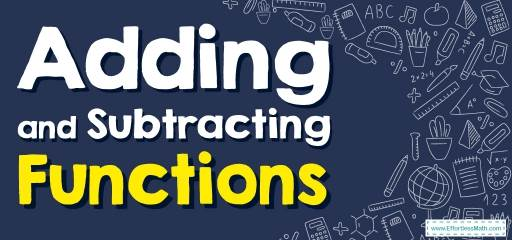# How to Add and Subtract Functions? (+FREE Worksheet!)

Learn how to add and subtract Functions by finding and combining like terms.## Step by step guide to Adding and Subtracting Functions

• Just like we can add and subtract numbers, we can add and subtract functions. For example, if we had functions $$f(x)$$ and $$g(x)$$, we could create two new functions: $$(f + g)(x)$$ and $$(f – g)(x)$$.
• When functions contain polynomials, find the like terms and combine them to add or subtract functions.
• When evaluating functions, substitute the value of the input and find the value of the function.

A Perfect Practice Workbook for Algebra I

### Adding and Subtracting Functions – Example 1:

$$g(a)=a-1, f(a)=a+5$$, Find: $$(g+f)(-1)$$

Solution:

$$(g+f)(a)=g(a)+f(a)$$, then: $$(g+f)(a)=a-1+a+5=2a+4$$
Substitute $$a$$ with $$-1: (g+f)(-1)=2a+4=2(-1)+4=-2+4=2$$

### Adding and Subtracting Functions – Example 2:

$$f(x)=3x-3, g(x)=x-5$$, Find: $$(f-g)(3)$$

Solution:

$$(f-g)(x)=f(x)-g(x)$$, then: $$(f-g)(x)=3x-3-(x-5)=3x-3-x+5 =2x+2$$
Substitute $$x$$ with $$3: (f-g)(3)=2x+2=2(3)+2=6+2=8$$

### Adding and Subtracting Functions – Example 3:

$$f(x)=2x+4, g(x)=x+3$$, Find: $$(f-g)(1)$$

Solution:

$$(f-g)(x)=f(x)-g(x)$$, then: $$(f-g)(x)=2x+4-(x+3) =2x+4-x-3=x+1$$
Substitute $$x$$ with $$1: (f-g)(1)=x+1=1+1=2$$

Best Algebra Prep Resource

### Adding and Subtracting Functions – Example 4:

$$g(a)=2a-1, f(a)=-a-4$$, Find: $$(g+f)(-1)$$

Solution:

$$(g+f)(a)=g(a)+f(a)$$, then: $$(g+f)(a)=2a-1-a-4=a-5$$
Substitute $$a$$ with $$-1: (g+f)(-1)=a-5=-1-5=-6$$

## Exercises for Adding and Subtracting Functions

### Perform the indicated operation.

1. $$\color{blue}{h(t) = 2t + 1, \\ g(t) = 2t + 2, \\ Find \ (h – g)(t)} \\\$$
2. $$\color{blue}{g(a) = – 3a – 3, \\ f(a) = a^2 + 5, \\ Find \ (g – f)(a)} \\\$$
3. $$\color{blue}{g(x) = 2x – 5, \\ h(x) = 4x + 5, \\ Find \ (g – h)(3)} \\\$$
4. $$\color{blue}{h(x) = 3x + 3, \\ g(x) = – 4x + 1, \\ Find \ (h + g)(10)} \\\$$
5. $$\color{blue}{f(x) = 4x – 3, \\ g(x) = x^3 + 2x, \\ Find \ (f – g)(4)} \\\$$
6. $$\color{blue}{h(n) = 4n + 5, \\ g(n) = 3n + 4, \\ Find \ (h – g)(n)}$$

1. $$\color{blue}{-1}$$
2. $$\color{blue}{–a^2 – 3a – 8}$$
3. $$\color{blue}{-16}$$
4. $$\color{blue}{-6}$$
5. $$\color{blue}{-59}$$
6. $$\color{blue}{n+1}$$

The Absolute Best Books to Ace Algebra

### What people say about "How to Add and Subtract Functions? (+FREE Worksheet!) - Effortless Math: We Help Students Learn to LOVE Mathematics"?

No one replied yet.

X
45% OFF

Limited time only!

Save Over 45%

SAVE $40 It was$89.99 now it is \$49.99